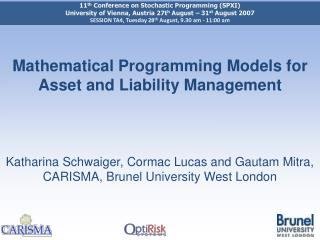Download PresentationMathematical Programming Models for Asset and Liability Management Katharina Schwaiger, Cormac Lucas and Gautam Mitra, C

# Mathematical Programming Models for Asset and Liability Management Katharina Schwaiger, Cormac Lucas and Gautam Mitra, C - PowerPoint PPT PresentationDownload Presentation## Mathematical Programming Models for Asset and Liability Management Katharina Schwaiger, Cormac Lucas and Gautam Mitra, C

- - - - - - - - - - - - - - - - - - - - - - - - - - - E N D - - - - - - - - - - - - - - - - - - - - - - - - - - -
##### Presentation Transcript

1. 11th Conference on Stochastic Programming (SPXI) University of Vienna, Austria 27th August – 31st August 2007 SESSION TA4, Tuesday 28th August, 9.30 am -11:00 am Mathematical Programming Models for Asset and Liability ManagementKatharina Schwaiger, Cormac Lucas and Gautam Mitra,CARISMA, Brunel University West London

2. Outline • Problem Formulation • Scenario Models for Assets and Liabilities • Mathematical Programming Models and Results: • Linear Programming Model • Stochastic Programming Model • Chance-Constrained Programming Model • Integrated Chance-Constrained Programming Model • Discussion and Future Work

3. Problem Formulation • Pension funds wish to make integrated financial decisions to match and outperform liabilities • Last decade experienced low yields and a fall in the equity market • Risk-Return approach does not fully take into account regulations (UK case) use of Asset Liability Management Models

4. Pensions: Introduction • Two broad types of pension plans: defined-contribution and defined-benefit pension plans • Defined-contribution plan: benefit depends on accumulated contributions at time of retirement • Defined-benefit plan: benefit depends on length of employment and final salary • We consider only defined-benefit pension plans

5. Pension Fund Cash Flows Sponsoring Company Figure 1: Pension Fund Cash Flows • Investment: portfolio of fixed income and cash

6. Mathematical Models • Different ALM models: • Ex ante decision by Linear Programming (LP) • Ex ante decision by Stochastic Programming (SP) • Ex ante decision by Chance-Constrained Programming • Ex ante decision by Integrated Chance-Constrained Programming • All models are multi-objective: (i) minimise deviations (PV01 or NPV) between assets and liabilities and (ii) reduce initial cash required

7. Asset/Liability under uncertainty • Future asset returns and liabilities are random • Generated scenarios reflect uncertainty • Discount factor (interest rates) for bonds and liabilities is random • Pension fund population (affected by mortality) and defined benefit payments (affected by final salaries) are random

8. Scenario Generation • LIBOR scenarios are generated using the Cox, Ingersoll, and Ross Model (1985) • Salary curves are a function of productivity (P), merit and inflation (I) rates • Pension Fund Population is determined using standard UK mortality tables

9. Salary Curve Example Salary Curves:

10. Fund Population Example Pension Fund Population:

11. Linear Programming Model • Deterministic with decision variables being: • Amount of bonds sold • Amount of bonds bought • Amount of bonds held • PV01 over and under deviations • Initial cash injected • Amount lent • Amount borrowed • Multi-Objective: • Minimise total PV01 deviations between assets and liabilities • Minimise initial injected cash

12. Linear Programming Model • Subject to: • Cash-flow accounting equation • Inventory balance • Cash-flow matching equation • PV01 matching • Holding limits

13. Linear Programming Model PV01 Deviation-Budget Trade Off

14. Stochastic Programming Model • Two-stage stochastic programming model with amount of bonds held , sold and bought and the initial cash being first stage decision variables • Amount borrowed , lent and deviation of asset and liability present values ( , ) are the non-implementable stochastic decision variables • Multi-objective: • Minimise total present value deviations between assets and liabilities • Minimise initial cash required

15. SP Model Constraints • Cash-Flow Accounting Equation: • Inventory Balance Equation: • Present Value Matching of Assets and Liabilities:

16. SP Constraints cont. • Matching Equations: • Non-Anticipativity:

17. Stochastic Programming Model Deviation-Budget Trade-off

18. Chance-Constrained Programming Model • Introduce a reliability level , where , which is the probability of satisfying a constraint and is the level of meeting the liabilities, i.e. it should be greater than 1 in our case • Scenarios are equally weighted, hence • The corresponding chance constraints are:

19. CCP Model Cash versus beta

20. CCP Model SP versus CCP frontier

21. Integrated Chance Constraints • Introduced by Klein Haneveld  • Not only the probability of underfunding is important, but also the amount of underfunding (conceptually close to conditional surplus-at-risk CSaR) is important Where is the shortfall parameter

22. ICCP Model SP versus ICCP frontier:

23. ICCP Model SP versus ICCP:

24. ICCP Model SP versus ICCP:

25. Discussion and Future Work Generated Model Statistics: * : Using CPLEX10.1 on a P4 3.0 GHz machine

26. Discussion and Future Work • Ex post Simulations: • Stress testing • In Sample testing • Backtesting

27. References • J.C. Cox, J.E. Ingersoll Jr, and S.A. Ross. A Theory of the Term Structure of Interest Rates, Econometrica, 1985. • R. Fourer, D.M. Gay and B.W. Kernighan. AMPL: A Modeling Language for Mathematical Programming. Thomson/Brooks/Cole, 2003. • W.K.K. Haneveld. Duality in stochastic linear and dynamic programming. Volume 274 of Lecture Notes in Economics and Mathematical Systems. Springer Verlag, Berlin, 1986. • W.K.K. Haneveld and M.H. van der Vlerk. An ALM Model for Pension Funds using Integrated Chance Constraints. University of Gröningen, 2005. • K. Schwaiger, C. Lucas and G. Mitra. Models and Solution Methods for Liability Determined Investment. Working paper, CARISMA Brunel University, 2007. • H.E. Winklevoss. Pension Mathematics with Numerical Illustrations. University of Pennsylvania Press, 1993.